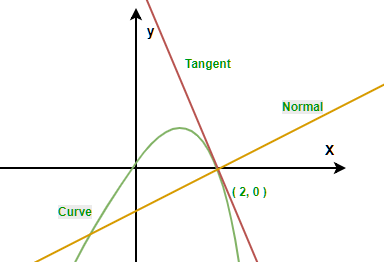# Find Tangent at a given point on the curve

• Last Updated : 14 Jun, 2022

Given a curve [ y = x(A – x) ], the task is to find tangent at given point (x, y) on that curve, where A, x, y are integers.
Examples:

```Input: A = 2, x = 2, y = 0
Output: y = -2x - 4
Since y = x(2 - x)
y = 2x - x^2 differentiate it with respect to x
dy/dx = 2 - 2x  put x = 2, y = 0 in this equation
dy/dx = 2 - 2* 2 = -2
equation  => (Y - 0 ) = ((-2))*( Y - 2)
=> y = -2x -4

Input: A = 3, x = 4, y = 5
Output: Not possible
Point is not on that curve```Approach:

1. First find if the given point is on that curve or not.
2. If the point is on that curve then, Find the derivative
3. Calculate the gradient of the tangent by Putting x, y in dy/dx.
4. Determine the equation of the tangent by substituting the gradient of the tangent and the coordinates of the given point into the gradient-point form of the straight line equation, where Equation of normal is Y – y = ( dy/dx ) * (X – x).

Below is the implementation of the above approach:

## C++

 `// C++ program for find Tangent``// on a curve at given point``  ` `#include ``using` `namespace` `std;``  ` `// function for find Tangent``void` `findTangent(``int` `A, ``int` `x, ``int` `y)``{``    ``// differentiate given equation``    ``int` `dif = A - x * 2;``  ` `    ``// check that point on the curve or not``    ``if` `(y == (2 * x - x * x)) {``  ` `        ``// if differentiate is negative``        ``if` `(dif < 0)``            ``cout << ``"y = "``                 ``<< dif << ``"x"` `<< (x * dif) + (y);``  ` `        ``else` `if` `(dif > 0)``  ` `            ``// differentiate is positive``            ``cout << ``"y = "``                 ``<< dif << ``"x+"` `<< -x * dif + y;``  ` `        ``// differentiate is zero``        ``else``            ``cout << ``"Not possible"``;``    ``}``}``  ` `// Driver code``int` `main()``{``    ``// declare variable``    ``int` `A = 2, x = 2, y = 0;``  ` `    ``// call function findTangent``    ``findTangent(A, x, y);``  ` `    ``return` `0;``}`

## Java

 `// Java program for find Tangent``// on a curve at given point``import` `java.util.*;``import` `java.lang.*;``import` `java.io.*;``  ` `class` `GFG``{``   ` `// function for find Tangent``static` `void` `findTangent(``int` `A, ``int` `x, ``int` `y)``{``    ``// differentiate given equation``    ``int` `dif = A - x * ``2``;``   ` `    ``// check that point on the curve or not``    ``if` `(y == (``2` `* x - x * x)) {``   ` `        ``// if differentiate is negative``        ``if` `(dif < ``0``)``            ``System.out.println( ``"y = "``                 ``+ dif + ``"x"` `+ (x * dif + y));``   ` `        ``else` `if` `(dif > ``0``)``   ` `            ``// differentiate is positive``            ``System.out.println( ``"y = "``                 ``+ dif + ``"x+"` `+ -x * dif + y);``   ` `        ``// differentiate is zero``        ``else``            ``System.out.println(``"Not possible"``);``    ``}``}``   ` `// Driver code``public` `static` `void` `main(String args[])``{``    ``// declare variable``    ``int` `A = ``2``, x = ``2``, y = ``0``;``   ` `    ``// call function findTangent``    ``findTangent(A, x, y);``   ` `} ``}`

## Python3

 `# Python3 program for find Tangent``# on a curve at given point``  ` `# function for find Tangent``def` `findTangent(A, x, y) :``  ` `    ``#  differentiate given equation``    ``dif ``=` `A ``-` `x ``*` `2``  ` `    ``#  check that point on the curve or not``    ``if` `y ``=``=` `(``2` `*` `x ``-` `x ``*` `x) :``  ` `        ``# if differentiate is negative``        ``if` `dif < ``0` `:``  ` `            ``print``(``"y ="``,dif,``"x"``,(x ``*` `dif) ``+` `(y))``  ` `        ``# differentiate is positive``        ``elif` `dif > ``0` `:``  ` `            ``print``(``"y ="``,dif,``"x+"``,``-``x ``*` `dif ``+` `y)``  ` `        ``# differentiate is zero``        ``else` `:``              ` `            ``print``(``"Not Possible"``)``              ` `   ``# Driver code    ``if` `__name__ ``=``=` `"__main__"` `:``  ` `    ``# declare variable``    ``A, x, y ``=` `2``, ``2``, ``0``  ` `    ``# call function findTangent``    ``findTangent(A, x, y)``                   ` `# This code is contributed by``# ANKITRAI1`

## C#

 `// C# program for find Tangent``// on a curve at given point``   ` `using` `System;``class` `GFG``{``     ` `// function for find Tangent``static` `void` `findTangent(``int` `A, ``int` `x, ``int` `y)``{``    ``// differentiate given equation``    ``int` `dif = A - x * 2;``     ` `    ``// check that point on the curve or not``    ``if` `(y == (2 * x - x * x)) {``     ` `        ``// if differentiate is negative``        ``if` `(dif < 0)``            ``Console.Write( ``"y = "``                 ``+ dif + ``"x"` `+ (x * dif + y)+``"\n"``);``     ` `        ``else` `if` `(dif > 0)``     ` `            ``// differentiate is positive``            ``Console.Write( ``"y = "``                 ``+ dif + ``"x+"` `+ -x * dif + y+``"\n"``);``     ` `        ``// differentiate is zero``        ``else``            ``Console.Write(``"Not possible"``+``"\n"``);``    ``}``}``     ` `// Driver code``public` `static` `void` `Main()``{``    ``// declare variable``    ``int` `A = 2, x = 2, y = 0;``     ` `    ``// call function findTangent``    ``findTangent(A, x, y);``     ` `}  ``}`

## PHP

 ` 0)``  ` `            ``// differentiate is positive``            ``echo` `"y = "``,``                ``\$dif` `, ``"x+"` `, -``\$x` `* ``\$dif` `+ ``\$y``;``  ` `        ``// differentiate is zero``        ``else``            ``echo` `"Not possible"``;``    ``}``}``  ` `// Driver code``  ` `// declare variable``\$A` `= 2;``\$x` `= 2;``\$y` `= 0;``  ` `// call function findTangent``findTangent(``\$A``, ``\$x``, ``\$y``);``  ` `// This code is contributed by Sachin``?>`

## Javascript

 ``

Output:

`y = -2x-4`

Time Complexity : O(1) ,as we are not using any loop.

Auxiliary Space : O(1) ,as we are not using any extra space.

My Personal Notes arrow_drop_up